# PSAT Math : Variables

## Example Questions

← Previous 1 3 4 5 6 7 8

### Example Question #1 : Variables

Factor the following variable

(x2 + 18x + 72)

(x + 6) (x + 12)

(x – 6) (x – 12)

(x – 6) (x + 12)

(x + 6) (x – 12)

(x + 18) (x + 72)

(x + 6) (x + 12)

Explanation:

You need to find two numbers that multiply to give 72 and add up to give 18

easiest way: write the multiples of 72:

1, 72

2, 36

3, 24

4, 18

6, 12: these add up to 18

(x + 6)(x + 12)

### Example Question #1 : Factoring

Factor 9x2 + 12x + 4.

(3x + 2)(3x – 2)

(3x – 2)(3x – 2)

(9x + 4)(9x + 4)

(9x + 4)(9x – 4)

(3x + 2)(3x + 2)

(3x + 2)(3x + 2)

Explanation:

Nothing common cancels at the beginning. To factor this, we need to find two numbers that multiply to 9 * 4 = 36 and sum to 12. 6 and 6 work.

So 9x2 + 12x + 4 = 9x2 + 6x + 6x + 4

Let's look at the first two terms and last two terms separately to begin with. 9x2 + 6x can be simplified to 3x(3x + 2) and 6x + 4 can be simplified into 2(3x + 2). Putting these together gets us

9x2 + 12x + 4

= 9x2 + 6x + 6x + 4

= 3x(3x + 2) + 2(3x + 2)

= (3x + 2)(3x + 2)

This is as far as we can factor.

### Example Question #2 : Factoring

If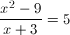, and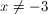, what is the value of?

–6

8

0

–8

6

8

Explanation:

The numerator on the left can be factored so the expression becomes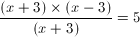, which can be simplified to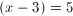Then you can solve forby adding 3 to both sides of the equation, so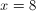### Example Question #1 : Factoring

Solve for x: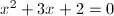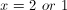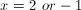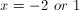Explanation:

First, factor.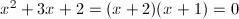Set each factor equal to 0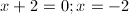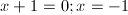Therefore,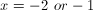### Example Question #1 : Factoring

When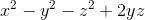is factored, it can be written in the form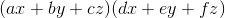, where,,,,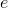, andare all integer constants, and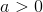.

What is the value of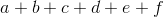?Explanation:

Let's try to factor x2 – y2 – z2 + 2yz.

Notice that the last three terms are very close to y2 + z2 – 2yz, which, if we rearranged them, would become y2 – 2yz+ z2. We could factor y2 – 2yz+ z2 as (y – z)2, using the general rule that p2 – 2pq + q2 = (p – q)2 .

So we want to rearrange the last three terms. Let's group them together first.

x2 + (–y2 – z2 + 2yz)

If we were to factor out a –1 from the last three terms, we would have the following:

x2 – (y2 + z2 – 2yz)

Now we can replace y2 + z2 – 2yz with (y – z)2.

x2 – (y – z)2

This expression is actually a differences of squares. In general, we can factor p2 – q2 as (p – q)(p + q). In this case, we can substitute x for p and (y – z) for q.

x2 – (y – z)= (x – (y – z))(x  + (y – z))

Now, let's distribute the negative one in the trinomial x – (y – z)

(x – (y – z))(x  + (y – z))

(x – y + z)(x + y – z)

The problem said that factoring x2 – y2 – z2 + 2yz would result in two polynomials in the form (ax + by + cz)(dx + ey + fz), where a, b, c, d, e, and f were all integers, and a > 0.

(x – y + z)(x + y – z) fits this form. This means that a = 1, b = –1, c = 1, d = 1, e = 1, and f = –1. The sum of all of these is 2.

### Example Question #1 : Variables

Factor and simplify: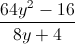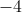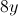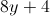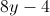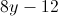Explanation: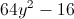is a difference of squares.

The difference of squares formula is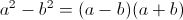.

Therefore,=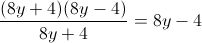.

### Example Question #3 : Factoring

Factor: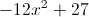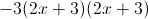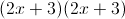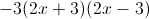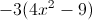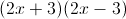Explanation:

We can first factor out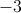:This factors further because there is a difference of squares:### Example Question #1 : Factoring Polynomials

What is a possible value for x in x2 – 12x + 36 = 0 ?

2

There is not enough information

6

–6

6

Explanation:

You need to factor to find the possible values for x. You need to fill in the blanks with two numbers with a sum of -12 and a product of 36. In both sets of parenthesis, you know you will be subtracting since a negative times a negative is a positive and a negative plus a negative is a negative

(x –__)(x –__).

You should realize that 6 fits into both blanks.

You must now set each set of parenthesis equal to 0.

x – 6 = 0; x – 6 = 0

Solve both equations: x = 6

### Example Question #41 : Variables

If r and t are constants and x2  +rx +6=(x+2)(x+t), what is the value of r?

6

7

5

It cannot be determined from the given information.

5

Explanation:

We first expand the right hand side as x2+2x+tx+2t and factor out the x terms to get x2+(2+t)x+2t. Next we set this equal to the original left hand side to get x2+rx +6=x2+(2+t)x+2t, and then we subtract x2  from each side to get rx +6=(2+t)x+2t. Since the coefficients of the x terms on each side must be equal, and the constant terms on each side must be equal, we find that r=2+t and 6=2t, so t is equal to 3 and r is equal to 5.

### Example Question #1 : Factoring Polynomials

Solve for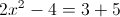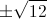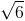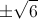Explanation:First, add 4 to both sides: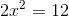Divide both sides by 2: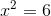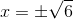← Previous 1 3 4 5 6 7 8

### All PSAT Math Resources